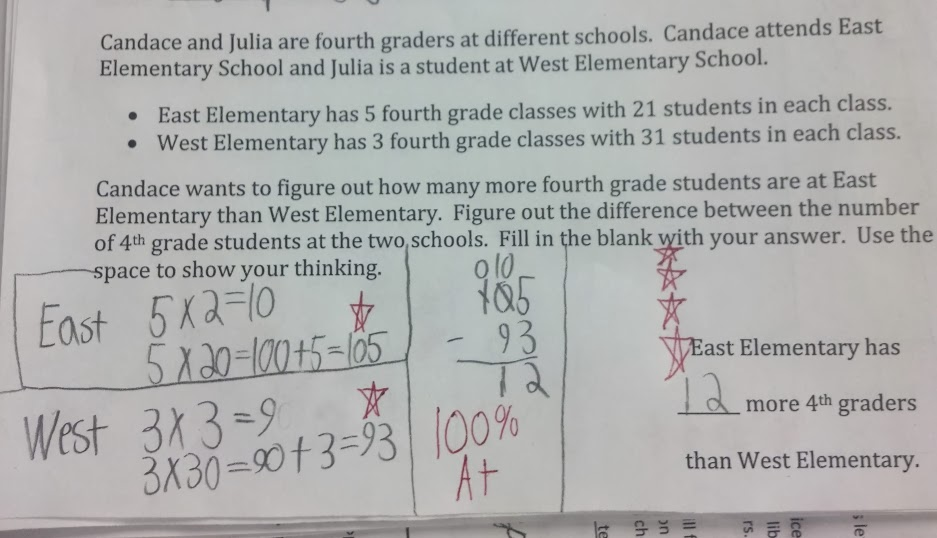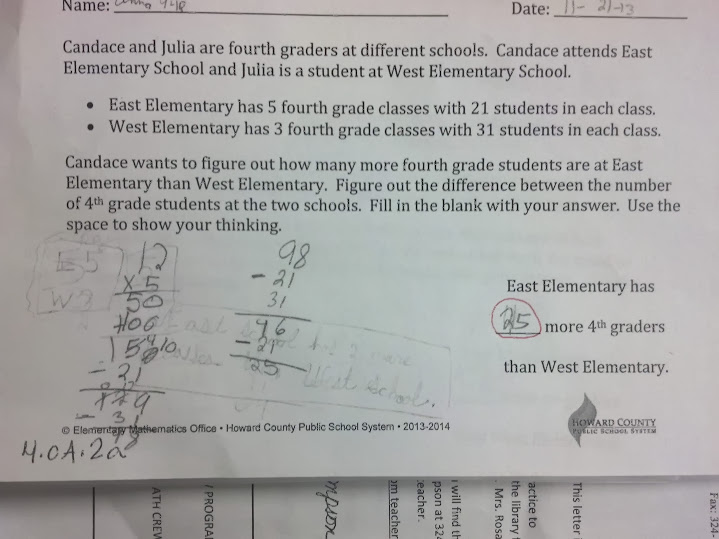# Making Sense of Remainders Day 2

4 teachers like this lesson
Print Lesson

## Objective

SWBAT investigate real world problems in which remainders are rounded, shared, or dropped.

#### Big Idea

Students work at developing an understanding of remainders in real life situations.

## Warm Up

5 minutes

Students begin this warm up with a formative assessment and review CCSS 4.OA.2.a.   Students use multiplication and subtraction in this multi-step word problem.  I do not require students to use multiplication in this assessment. They can also use repeated addition.

A sample of the problem is below.  This problem came from the Howard County Math Wiki site for fourth grade.  I used this problem as a review.  Based on student results, I make small re-teaching groups as necessary to ensure students get continued scaffolds and differentiation.You can see in this photo, a student's work with an incorrect answer.  You can see this student is struggling with many concepts.  The student has multiplied incorrectly and also doesn't appear to be able to make sense of the problem.  It is clear that the student views this problem as a subtraction problem, but does not understand what numbers to subtract or how to find the number of fourth grade students at each school. This student is marked on my re-teach list for several different areas, but will start with reviewing the skill of multiplication.## Concept development

45 minutes

It often takes a jump in understanding for students to apply the procedural algorithm of division with remainders to real-world situations where remainders are encountered. A child who can easily calculate 40 divided by 6 = 6R4 will too often state 6R4 as the answer to the number of cars necessary to transport 40 children to a baseball game if 6 children can fit in each car.

The problems in this lesson will first review the concept of division as proportional reasoning involving equal shares and then they will lead children to discover the three usual ways of dealing with remainders in real life: they are either used to round up to the next whole number, they are dropped and discarded, or they are split evenly among the participants.

I start this lesson by reading the story, located in the resource section, Round Up.  I have students sit on the floor with whiteboards.  As I read the story, I stop at specific spots that allow students to find the quotient and remainder before the characters in the story give the answer.  The story helps emphasize the concept of rounding the remainder due to the situation in the story.

After the story, students work with their learning partner and table mates to work on the division problem worksheet.  I have students fold a separate piece of paper into thirds and have them complete the problems on this separate paper.  I only do this to cut down on printing costs.  I prefer to have each student have their own paper, but this is a good alternative.

Students work to finish the first three problems on the worksheet. After they have a period of time to work, and when it looks like most partners are finished with the three problems, we talk about the answers together.

Note: At this point in the year, many of my students use multiplication to figure out the answer or a grouping strategy. I specifically choose to do this assignment before I've taught any procedures or strategies because I think students come to school with many strategies that work. By knowing that many will use multiplication to get their answer, I can then lead discussions about the relationships between multiplication and division.

Listen in as this student explains why she would round the remainder in a division situation.